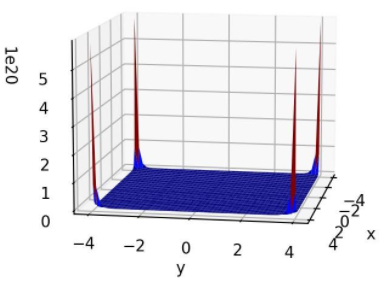top of page
Search

# Brown Function

Updated: Jul 18, 2021Mathematical DefinitionInput Domain

Brown Function is usually evaluated for the range: -1≤ xi≤ 4 for i=1,….,n. This function is smooth.

Global Minima

The function has one global minimum f (x*) = =0 at x*=(0,...,0)

Description and Features

Brown Function is a unimodal optimization problem. It is scalable and is defined on n- dimensional space.

Python Implementation

```% Please forward any comments or bug reports in chat
Copyright 2021. INDUSMIC PRIVATE LIMITED.THERE IS NO WARRANTY, EXPRESS OR IMPLIED. WE DO NOT ASSUME ANY LIABILITY FOR THE USE OF THIS PROGRAM. If software is modified to produce derivative works, such modified software should be clearly marked. Additionally, user can redistribute it and/or modify it under the terms of the GNU General Public License. This program is distributed in the hope that it will be useful, but WITHOUT ANY WARRANTY. See the GNU General Public License for more details.
% for any support connect with us on help.indusmic@gmail.com
% Author: Vanshita Tripathi

import matplotlib.pyplot as plt
import matplotlib as mpl
from mpl_toolkits import mplot3d
from mpl_toolkits.mplot3d import Axes3D
import numpy as np
from numpy import*
%matplotlib notebook
plt.rcParams['figure.figsize'] = (6,4)
plt.rcParams['figure.dpi']=150
fig=plt.figure()
axis = fig.gca(projection= '3d')

def f(x1,x2):
a= (x1*x1)**(x2*x2 + 1) + (x2*x2)**(x1*x1 +1)
return a
x1= linspace(4,-4)
x2= linspace(4,-4)
X1,X2= meshgrid(x1,x2)
ax.plot_surface(X1,X2,f(X1,X2), cmap='jet')
ax.set_xlabel('x')
ax.set_ylabel('y')
axis.view_init(10,10)
plt.show```

References:

 Jamil, Momin, and Xin-She Yang. "A literature survey of benchmark functions for global optimization problems." International Journal of Mathematical Modelling and Numerical Optimization 4.2 (2013): 150-194.